# RD Sharma Solutions Class 9 Chapter 12 Herons Formula

Read RD Sharma Solutions Class 9 Chapter 12 Herons Formula below, students should study RD Sharma class 9 Mathematics available on Studiestoday.com with solved questions and answers. These chapter wise answers for class 9 Mathematics have been prepared by teacher of Grade 9. These RD Sharma class 9 Solutions have been designed as per the latest NCERT syllabus for class 9 and if practiced thoroughly can help you to score good marks in standard 9 Mathematics class tests and examinations

Exercise 12.1

Question 1: Find the area of a triangle whose sides are respectively 150 cm, 120 cm and 200 cm.
Solution:
It is given that,
a = 150 cm
b = 120 cm
c = 200 cmQuestion 2: Find the area of a triangle whose sides are respectively 9 cm, 12 cm and 15 cm.
Solution:
a = 9 cm
b = 12 cm
c = 15 cm

s = ((a+b+c))/2
s = ((9 + 12 + 15))/2
s = 18 cm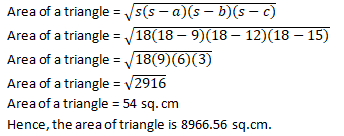Question 3: Find the area of a triangle two sides of which are 18 cm and 10 cm and the perimeter is 42 cm.
Solution:
It is given that,
a = 18 cm
b = 10 cm
Perimeter = 42 cm
c be the third side of the triangle.
We know, perimeter = a + b + c
S = 42/2
S = 21
S = ((a+b+c))/2
Put the value of s, we get
21 = ((18 + 10 + c))/2
42 = 28 + c
c = 14 cm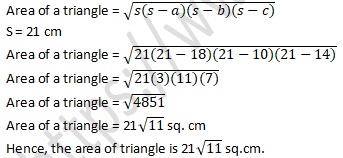Question 4:  In a triangle ABC, AB = 15cm, BC = 13cm and AC = 14cm. Find the area of triangle ABC and hence its altitude on AC.
Solution:
AB = a
BC = b
AC = c
a = 15 cm
b = 13 cm
c = 14 cm
s = ((a+b+c))/2
s = ((15 + 13 + 14))/2
s = 42/2
s = 21cm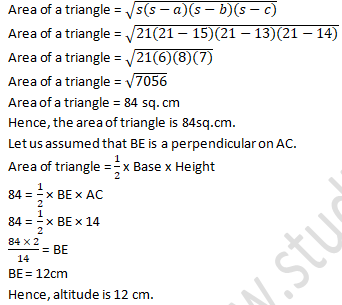Question 5: The perimeter of a triangular field is 540 m and its sides are in the ratio 25 : 17 : 12. Find the area of the triangle.
Solution:
Let us assumed that the sides of a triangle
a = 25x
b = 17x
c = 12x
Perimeter of triangle = 540 cm
2s = a + b + c
a + b + c = 540cm
25x + 17x + 12x = 540cm
54x = 540cm
54x = 540/54cm
x = 10cm
The sides of a triangle are
a = 250 cm
b = 170 cm
c = 120 cm
S = ((a+b+c))/2
S = 540/2
S = 270cmQuestion 6: The perimeter of a triangle is 300 m. If its sides are in the ratio of 3: 5: 7. Find the area of the triangle.
Solution:
Let us assumed that the sides of a triangle
a = 3x
b = 5x
c = 7x
Perimeter = a + b + c
300 = a + b + c
300 = 3x + 5x + 7x
300 = 15x
x = 20 m
So, the sides are
a = 60 m
b = 100 m
c = 140 m
S = ((a+b+c))/2
S = ((60+100+140))/2
S = 300/2
S = 150cm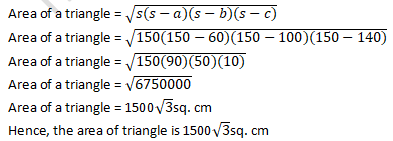Question 7:  The perimeter of a triangular field is 240dm. If two of its sides are 78dm and 50dm, find the length of the perpendicular on the side of length 50dm from the opposite vertex.
Solution:
It is given that,
AB = a = 78 dm
BC = b = 50 dm
Perimeter = 240 dm
AB + BC + AC = 240 dm
78 + 50 + AC = 240
AC = 240 - 78 - 50
AC = 112 dm
AC = c = 112 dm
s = (a + b + c)/2
s = (78 + 50 + 112)/2
s = 240/2dm
s = 120dm
Area of a triangle = √(s(s-a)(s-b)(s-c))Question 8:  A triangle has sides 35 cm, 54 cm, 61 cm long. Find its area. Also, find the smallest of its altitudes?
Solution:
It is given that given,
a = 35 cm
b = 54 cm
c = 61 cm
Perimeter 2s = a + b + c
s = (35 + 54 + 61)/2
s = 150/2
s = 75cm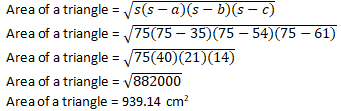Longest side = 61 cm
Area of the triangle = 1/2 × H × B
939.14 = 1/2 × H × 61
939.14 cm2 = 1/2 × H × 61
(939.14×2)/61   = H
H = 30.79 cm
Thus, the length of the smallest altitude is 30.79 cm

Question 9:  The lengths of the sides of a triangle are in a ratio of 3: 4: 5 and its perimeter is 144 cm. Find the area of the triangle and the height corresponding to the longest side?
Solution:
Let us assumed that the sides of a triangle
a = 3x
b = 4x
c = 5x
Perimeter = a + b + c
144 = 3x + 4x + 5x
144 = 12x
144/12 = x
x = 12 cm
So, the respective sides are
a = 3 × 12 = 36 cm
b = 4 × 12 = 48 cm
c = 5 × 12 = 60 cm
s = (a + b + c)/2
s = 144/2
s = 72cm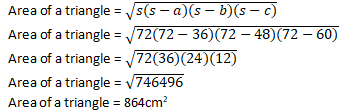Longest side = 60 cm
Area of the triangle = 1/2 × h × 60
864 cm2 = 1/2 × h × 60
(864 × 2)/60 = h
h = 28.8 cm
Thus the length of the smallest altitude is 28.8 cm

Question 10: The perimeter of an isosceles triangle is 42 cm and its base is 3/2 times each of the equal side. Find the length of each of the triangle, area of the triangle and the height of the triangle.
Solution:
Let the two sides of an isosceles triangle be x and x.
Third side ‘base’ = 3/2x
Perimeter = a + b + c
42 = x + x + 3/2x
42 = 2x + 3/2x
42 = (4x + 3x)/2
42 = 7x/2
(42 × 2)/7 = x
6×2 = x
12 = x
So, the sides are
a = x = 12 cm
b = x = 12 cm
c = 3/2×12 = 18 cm
s = (a + b + c)/2
s = (12 + 12 + 18)/2
s = 42/2
s = 21cmQuestion 11: Find the area of the shaded region in fig. below.Solution:
Area of the shaded region = Area of ΔABC − Area of ΔADB
It is given that,
BD =16 cm
AB2 = 122 + 162
AB2 = 400cm2
AB2 = √(400cm2 )
AB = 20 cm
Area of a triangle = 1/2 × AD × BD
Area of a triangle = 1/2 × 12 × 16
Area of a triangle = 96 cm2
In ∆ABC,
S = (AB + BC + CA)/2
S = (52 + 48 + 20)/2
S = 120/2
S = 60 cmExercise 12.2

Question 1: Find the area of the quadrilateral ABCD in which AB = 3 cm, BC = 4 cm, CD = 4 cm, DA = 5 cm and AC = 5 cm.
Solution:∆ABC is a right-angled triangle
Area of ∆ABC = 1/2  x Base x Height
Area of ∆ABC = 1/2 × AB × BC
Area of ∆ABC = 1/2×3 × 4
Area of ∆ABC = 6 cm2
From Pythagoras theorem
AC2 = AB2 + BC2
AC2 = (3)2 + (4)2
AC2 = 9 + 16
AC2 = 25
AC = √25
AC = 5cm
s = (a + b + c)/2
s = (5 + 4 + 5)/2
s = 14/2
s = 7cm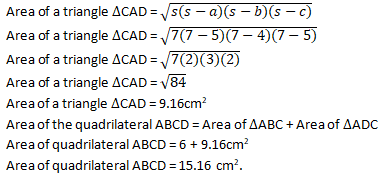Question 2:  The sides of a quadrilateral field, taken in order are 26m, 27m, 7m, and 24m respectively. The angle contained by the last two sides is a right angle. Find its area.
Solution: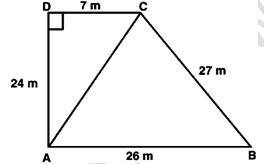It is given that,
AB = 26m
BC = 27m
CD = 7m
DA = 24m
AC is the diagonal joined at A to C point.
From Pythagoras theorem;
AC2 = (24)2 + (7)2
AC2 = 576 + 49
AC2 = 625
AC = √625
AC = 25cm
s = (a + b + c)/2
s = (25 + 26 + 27)/2
s = 78/2
s = 39cmArea of right triangles △ADC = 1/2 × Base × Height
Area of right triangles △ADC = 1/2 x 7 x 24
Area of right triangles △ADC = 84 m2

Area of rectangular field ABCD = Area of △ABC + Area of △ADC
Area of rectangular field ABCD = 291.84 m2 + 84 m2
Area of rectangular field ABCD = 375.8 m2

Question 3:  The sides of a quadrilateral, taken in order as 5, 12, 14, 15 meters respectively, and the angle contained by first two sides is a right angle. Find its area.
Solution: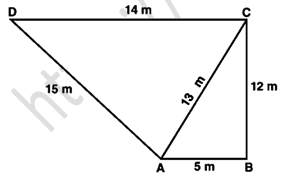It is given that,
AB = 5 m
BC = 12 m
CD =14 m
DA = 15 m
Join the diagonal AC.
Area of △ABC = 1/2 × AB × BC
Area of △ABC = 1/2 × 5 × 12
Area of △ABC = 30m2
In △ABC
From Pythagoras theorem,
AC2 = AB2 + BC2
AC2 = (5)2 + (12)2
AC2 = 25 + 144
AC2 = 169
AC = 13
s = (a + b + c)/2
s = (13 + 14 + 15)/2
s = 42/2
s = 21cm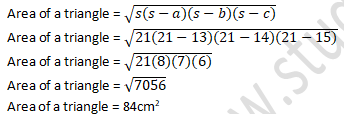Area of quadrilateral ABCD = Area of △ABC + Area of △ADC
Area of quadrilateral ABCD = 30 + 84 m2
Area of quadrilateral ABCD = 114 m2

Question 4:  A park in the shape of a quadrilateral ABCD, has ∠C = 90°, AB = 9 m, BC = 12 m, CD = 5 m, AD = 8 m. How much area does it occupy?
Solution: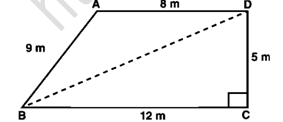It is given that,
AB = 9 m
BC = 12 m
CD = 5 m
DA = 8 m.
BD is a diagonal of ABCD.
In right ∆BCD,
From Pythagoras theorem;
BD2 = BC2 + CD2
BD2 = 122 + 5
BD2 = 144 + 25
BD2 = 169
BD = 13m
Area of ∆BCD = 1/2 × BC × CD
Area of ∆BCD = 1/2 × 12 × 5
Area of ∆BCD = 30m2
In △ABD,
s = (a + b + c)/2
s = (9 + 8 + 13)/2
s = 30/2
s = 15m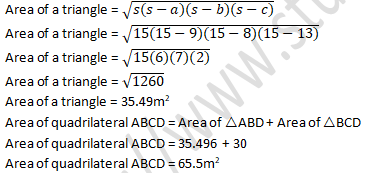Question 5:  Two parallel sides of a trapezium are 60 m and 77 m and the other sides are 25 m and 26 m. Find the area of the trapezium.
Solution:It is given that,

AB = 77m

CD = 60 m

BC = 26m

AE and CF are diagonals.

DE and CF are two perpendiculars on AB.

DC = EF = 60 m

Let us assumed that, AE = x

BF = 77 – (60 + x)

BF = 17 – xQuestion 6: Find the area of a rhombus whose perimeter is 80 m and one of whose diagonal is 24 m.
Solution:It is given that perimeter of a rhombus = 80m
Let the sides will be ‘a’
Perimeter of a rhombus = 4 × side
80 = 4 × a
80/4 = a
20 = a
Diagonal AC = 24m
OA = 1/2 × AC
OA = 1/2 × 24
OA = 12
In △AOB,
OB2 = AB2 − OA
OB2  = 202 −12
OB2  = 400 – 144
OB2  = 256
OB2  = √256
OB = 16
Since diagonal of rhombus bisect each other at 90 degrees.
And OB = OD
BD = 2 × OB
BD = 2 x 16m
BD = 32m
Area of rhombus = 1/2 × BD × AC
Area of rhombus = 1/2  × 32 × 24
Area of rhombus = 384m2

Question 7: A rhombus sheet, whose perimeter is 32 m and whose diagonal is 10 m long, is painted on both the sides at the rate of Rs 5 per m2. Find the cost of painting.
Solution:It is given that, Perimeter of a rhombus = 32 m
Let the side will be a.
Perimeter of a rhombus = 4 × side
32 = 4 × Side
32/4 = Side
8m = Side
So the side of a rhombus is 8.
AC = 10m
OA = 1/2 × AC
OA = 1/2 × 10
OA = 5m
In ∆AOB,
From Pythagoras theorem;
OB2 = AB2 − OA2
OB2  = 82 – 52
OB2  = 64 – 25
OB2  = 39
OB = √39m
BD = 2 x OB
BD = 2√39 m
Area of the sheet = 1/2 × BD × AC
Area of the sheet = 1/2 x (2√39 × 10)
Area of the sheet = 10√39
Area of the sheet is 10√39 m2
Cost of printing on both sides of the sheet, Rs. 5 per m2
Cost of printing = Rs. 2 x (10√39 x 5)
Cost of printing = Rs. 625

Question 8:  Find the area of the quadrilateral ABCD in which AD = 24 cm, ∠BAD = 90° and BCD forms an equilateral triangle whose each side is equal to 26 cm. [Take √3 = 1.73]
Solution:It is given that, ABCD is a quadrilateral
BCD is an equilateral triangle
BC = CD = BD = 26 cm
BA2 = 262 - 242
BA2 = 676 - 576
BA = √100
BA = 10 cm

Area of the ∆BAD = 1/2 × 10 × 24
Area of the ∆BAD = 120cm

Area of the equilateral triangle = √3/4 × side
Area of the equilateral triangle BCD = √3/4 × 26
Area of the equilateral triangle BCD = 292.37 cm2

The area of quadrilateral ABCD = Area of triangle BAD + Area of the triangle BCD
The area of quadrilateral ABCD = 120 + 292.37
The area of quadrilateral ABCD = 412.37 cm2

Question 9:  Find the area of quadrilateral ABCD in which AB = 42 cm, BC = 21 cm, CD = 29 cm, DA = 34 cm and the diagonal BD = 20 cm.
Solution: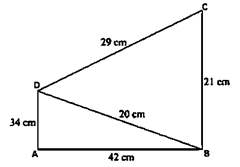It is given that,
AB = 42 cm
BC = 21 cm
CD = 29 cm
DA = 34 cm
The diagonal BD = 20cm
In ∆ABD
s = (a + b + c)/2
s = (34 + 42 + 20)/2
s = 96/2
s = 48 cm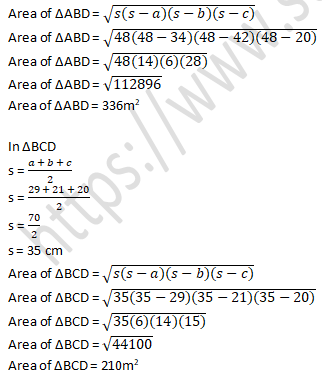Area of quadrilateral ABCD = Area of triangle ABD + Area of triangle BCD
Area of quadrilateral ABCD = 336 + 210
Area of quadrilateral ABCD = 546 cm2

Question 10: Find the perimeter and the area of the quadrilateral ABCD in which AB = 17 cm, AD = 9 cm, CD = 12 cm, AC = 15 cm and angle ACB = 90°.
Solution:It is given that,
AB = 17 cm
CD = 12 cm
AC = 15 cm
∠ACB = 90°
BC2 = AB2 − AC2
BC2 = 172 − 152
BC2 = 289 − 225
BC = √64
BC = 8cm
In ∆ABC
Area of ∆ABC = 1/2 × AC × BC
Area of ∆ABC = 1/2 × 8 × 15
Area of ∆ABC = 60 cm2

In ∆ACD
s = (a + b + c)/2
s = (15 + 12 + 9)/2
s = 36/2
s = 18 cmArea of quadrilateral ABCD = Area of triangle ABC + Area of triangle ACD
Area of quadrilateral ABCD = 60 cm2 + 54 cm2
Area of quadrilateral ABCD = 114 cm2

Question 11:  The adjacent sides of a parallelogram ABCD measure 34 cm and 20 cm, and the diagonal AC measures 42 cm. Find the area of parallelogram.
Solution: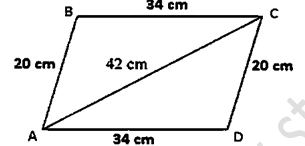Area of triangle ABC = Area of triangle ADC
Area of the parallelogram = Area of triangle ADC + Area of triangle ABC
Area of the parallelogram = 2 × (Area of triangle ABC)
Area of triangle ABC
s = (34 + 20 + 42 )/2
s = 96/2
s = 48 cmArea of parallelogram ABCD = 2 × (Area of triangle ABC)
Area of parallelogram ABCD = 2 × 336 cm2
Area of parallelogram ABCD = 672 cm2

Question 12:  Find the area of the blades of the magnetic compass shown in figure given below:
Solution:Area of the blades of magnetic compass = Area of triangle ADB + Area of triangle CDB
s = (5 + 1 + 5 )/2
s = 11/2
s = 5.5 cm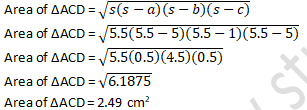Area of triangle ADB = Area of triangle CDB
Area of the blades of the magnetic compass = 2 × area of triangle ADB
Area of the blades of the magnetic compass = 2 × 2.49
Area of the blades of the magnetic compass = 4.98 cm2

Question 13:  A hand fan is made by sticking 10 equal size triangular strips of two different types of paper as shown in the figure. The dimensions of equal strips are 25 cm, 25 cm and 14 cm. Find the area of each type of paper needed to make the hand fan.Solution:
It is given that,
The sides of AOB
AO = 25 cm
OB = 25 cm
BA = 14 cm
Area of each strip = Area of triangle AOB
In ∆AOB
s = (25 + 14 + 25 )/2
s = 64/2
s = 32 cm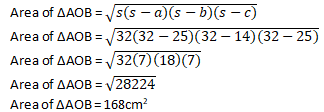Area of each type of paper needed to make a fan = 5 × Area of triangle AOB
Area of each type of paper needed to make a fan = 5 × 168 cm2
Area of each type of paper needed to make a fan = 840 cm2

Question 14:  A triangle and a parallelogram have the same base and the same area. If the sides of the triangle are 13 cm, 14 cm and 15 cm and the parallelogram stands on the base 14 cm, find the height of a parallelogram.

Solution:
The sides of the triangle DCE are
DC = 15 cm,
CE = 13 cm,
ED = 14 cm

s = (15 + 13 + 14 )/2
s = 42/2
s = 21cm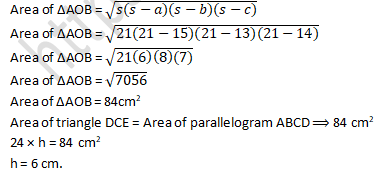Exercise VSAQs

Question 1: Find the area of a triangle whose base and altitude are 5 cm and 4 cm respectively.
Solution:
It is given that the Base of a triangle = 5cm and altitude = 4 cm
Area of triangle = 1/2 x base x altitude
Area of triangle = 1/2 x 5 x 4
Area of triangle = 10cm2

Question 2:  Find the area of a triangle whose sides are 3 cm, 4 cm and 5 cm respectively.
Solution:
s = (3+4+5)/2
s = 12/2
s = 6cm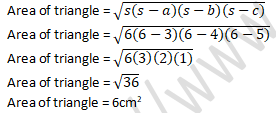Question 3: Find the area of an isosceles triangle having the base x cm and one side y cm.
Solution:Question 4:  Find the area of an equilateral triangle having each side 4 cm.
Solution:
Side of an equilateral triangle = 4 cm
Let the side will be ‘a’
Area of an equilateral triangle = √3/4 × side²
Area of an equilateral triangle =  √3/4 × 4²
Area of an equilateral triangle = 4√3cm2

Question 5: Find the area of an equilateral triangle having each side x cm.
Solution:
Side of an equilateral triangle = x cm
Area of an equilateral triangle = √3/4 × side²
Area of an equilateral triangle =  √3/4 × x²
Area of an equilateral triangle = (√3 x)/4cm2